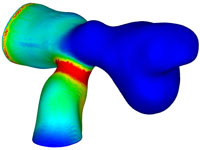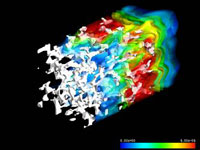# Lattice Boltzmann CFD

The Lattice Boltzmann (LB) method is based on the discrete simulation of a fluid. The method allows a highly efficient vector/parallel HPC implementation and is particularly suited for multi-physics complex-flow studies.### Method

The propagation and interaction of fluid particles is simulated via the time evolution of a particle distribution function.
This time- and space discrete Boltzmann-type equation with a linear collision term (the Lattice Boltzmann Equation) simulates a fluid flow governed by the Navier-Stokes equations.

### Computational efficiency

Close to linear scaling on massively parallel super-computers as well as more than 50% of an NEC SX vector super-computer's peak performance is reached with state of the art implementations. For complex flows this method is often more efficient than Navier-Stokes based FE/FM codes.### Literature

More information about my research on Lattice Boltzmann CFD and further literature can be found in my Ph.D. thesis "Simulation of complex flows and multi-physics with the Lattice-Boltzmann method", available for download at the Digital Academic Repository of the University of Amsterdam (all pictures in this page are from my thesis).

A list of textbooks on the Lattice Boltzmann method can be found here.

### Free software

A very simple implementation of the LB method is my teaching code anb. For larger simulations I recommend having a look at the software package Palabos.